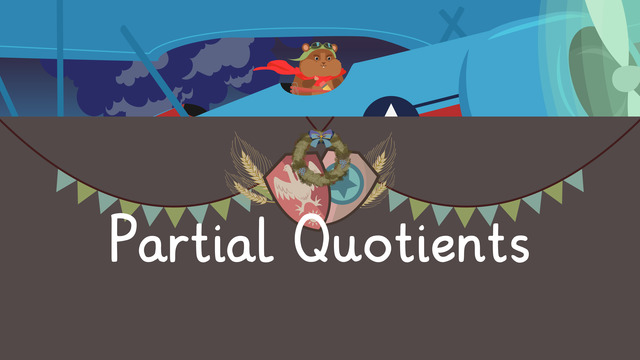# Long Division Using Partial QuotientsRating

Give us a rating!
The authorsTeam Digital
Long Division Using Partial Quotients
CCSS.MATH.CONTENT.4.NBT.B.6

## Basics on the topicLong Division Using Partial Quotients

How do you solve long division using partial quotients? Find out in this video!

### TranscriptLong Division Using Partial Quotients

The victory in the Great Beetle War would not have been possible without the help of hometown heroes like Airman Squeaks, who used the strategy of partial quotients to help deliver groups of supplies behind enemy lines. Another method for solving long-division problems is to use partial quotients. This method divides a problem into smaller, more manageable parts using multiples of ten or simple numbers. We'll use Airman Squeaks' supply deliveries to demonstrate how to divide three and four-digit numbers. He has four hundred thirty-two corn kernels to take to six battalions. We need to calculate how many kernels would be given to each. We set up the problem exactly as we would with the traditional long-division method. First, estimate how many times six goes into four hundred thirty-two. Ask yourself, what multiple of ten can we multiply by six to get close to this number? Six times seventy equals four hundred and twenty. Seventy is a partial quotient and goes here. Four hundred twenty is known as the partial dividend and is written here. Now we subtract the partial dividend from the original dividend. Subtract four hundred thirty-two from four hundred twenty to get twelve. Now ask ourselves, what do we multiply six by to get twelve? The partial quotient two goes here, and the partial dividend twelve goes here. We subtract again and get zero. We add the partial quotients to get the final answer. Seventy-two. Airman Squeaks will deliver seventy-two corn kernels to each battalion. He must also ensure that the troops are adequately supplied with insect repellent. He has seven hundred sixty-five cans that need to be delivered to five different locations. We set up the problem like this. What multiple of ten can we multiply five by to get close to seven hundred, sixty-five? Although there are many multiples we could choose, here we can say five times one hundred equals five hundred. What do we get when we subtract five hundred from seven hundred sixty-five? Two hundred, sixty-five. What multiple of ten can we multiply five by to get close to two hundred sixty-five? Five times fifty equals two hundred fifty. Subtract again. What is left? Fifteen. How many groups of five are in fifteen? Three. Three times five is fifteen. What is the sum of the partial quotients? One hundred fifty-three. Finally, Airman Squeaks had to deliver two thousand, three hundred sixty-seven sticks to build three barracks. How many sticks would each one receive? Pause the video and solve this one on your own and press play when you are ready to review it. Although there are many ways to begin multiplying this problem by using different multiples, we chose the largest number that would get us the closest. Three times seven hundred is two thousand one hundred. After we subtract, we have two hundred sixty-seven. Three times eighty gives us two hundred forty. We are left with twenty-seven. And three times nine equals twenty-seven. The sum of the partial quotients is seven hundred eighty-nine. While our hero returns from his daring mission, let's review. Remember, another method for solving long-division problems is to use partial quotients. This method divides a problem into smaller, more manageable parts using multiples of ten or simple numbers. Using multiples of ten or other simple numbers, we estimate how many times the divisor goes into the dividend. Airman Harry Squeaks has returned from a daring mission deep in enemy territory after weeks of anticipation. Once again, our valiant airmen have risked it all for our freedom.